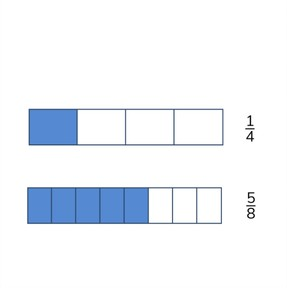Finding common denominators

# Finding common denominators

I can find common denominators8,000 schools use Gynzy92,000 teachers use Gynzy1,600,000 students use Gynzy

## General

Students learn to find a common denominator for fractions.

## Common core standard(s)

CCSS.Math.Content.4.NF.B.3a
CCSS.Math.Content.4.NF.A.2

## Relevance

Discus with students that it is important to be able to find a common denominator so that you can easily calculate with fractions.

## Introduction

Students read the fraction bar and name the fraction that it represents. Next they do the same with the fraction circle.

## Development

Show students that a fraction is made with a numerator and a denominator. To make fractions have a common denominator, you must make sure that the denominators of both fractions are the same. You can do this by multiplying or dividing one or both of the fractions. Show, using 4//16 that you can divide by 2 to get 2//8. Or how you can multiply 3//8 by 2 to get 6//16. Using the following problem with a fraction bar, check that students can convert 1//4 to eighths. Have students repeat this with the next two problems. If you have a fraction which must be converted to a specific denominator, then you divide to reach that denominator. The numerator must also be divisible by that same number. To demonstrate, show 4//8, 9//12, and 6//8. Explain that you can divide the first and last fractions by 2 to get fourths. The fraction 9//12 will have to be divided by 3 to get fourths. So you must also divide the numerator (9) by 3. Use the next problem to check that students are able to change their fractions to fifths and fortieths. Next, explain how students can find a common denominator for 2 fractions without knowing which denominator they must use. Tell students that you can multiply the denominators together to find the denominator you should use. Ask students to solve the following two problems to check their understanding. Students finally drag the fractions to the correct denominators.

Check that students are able to find common denominators by asking teh following questions:
- What are fractions with common denominators?
- How do you make fractions have a common denominator?

## Guided practice

Students are first given an exercise in which students must match the denominator of the visual given. Then students must determine a common denominator without visual support. Finally students are given multiple fractions to find a common denominator for.

## Closing

Discuss with students the importance of finding a common denominator with fractions. To close, have students practice with a set of three problems which can be solved individually or in groups/pairs.

## Teaching tips

Students who have difficulty finding the common denominator for fractions can first practice simplifying fractions. This can be visually supported by the use of a fraction circle, fraction bar or fraction support.

## Instruction materials

Optional: fraction bar, fraction circle, fraction manipulatives

### The online teaching platform for interactive whiteboards and displays in schools

• Save time building lessons

• Manage the classroom more efficiently

• Increase student engagement